### Home > CALC > Chapter 2 > Lesson 2.1.1 > Problem2-12

2-12.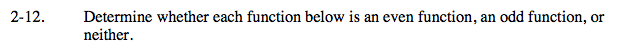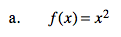Definition of an even function: f(a) = f(−a)
Definition of an odd function: f(−a) = −f(a)

f(x) = x²
f(a) = (a)²= a²
f(−a) = (−a)²= a².......... f(a) = f(−a) it's even!
f(a) = −(a)²= −a².............f(−a) ≠ −f(a) it's NOT odd.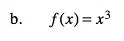Refer to hint and steps in part (a).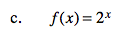Refer to hint and steps in part (a).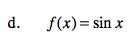Even functions are symmetrical over the y-axis.
Odd functions are symmetrical about the origin.

Sketch a graph of sinx and describe the symmetry.